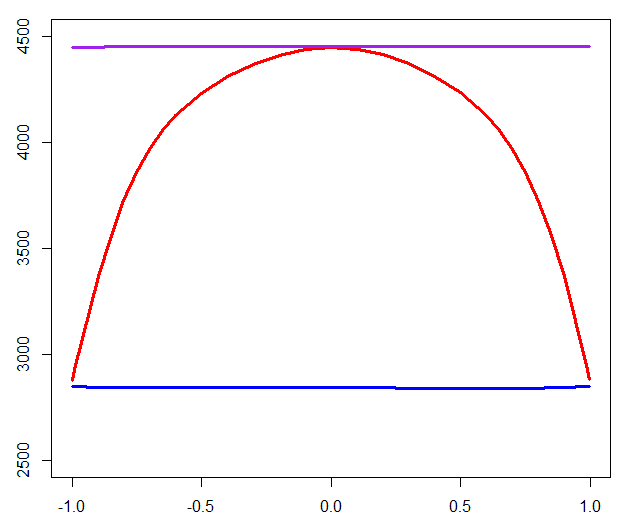Want to share your content on R-bloggers? click here if you have a blog, or here if you don't.

Variable importance graphs are great tool to see, in a model, which variables are interesting. Since we usually use it with random forests, it looks like it is works well with (very) large datasets. The problem with large datasets is that a lot of features are ‘correlated’, and in that case, interpretation of the values of variable importance plots can hardly be compared. Consider for instance a very simple linear model (the ‘true’ model, used to generate data)

Here, we use a random forest to model the relationship between the features, but actually, we consider another feature – not used to generate the data – , that is correlated to . And we consider a random forest on those three features, .

In order to get some more robust results, I geneate 100 datasets, of size 1,000.

```library(mnormt)

impact_correl=function(r=.9){
nsim=10
IMP=matrix(NA,3,nsim)
n=1000
R=matrix(c(1,r,r,1),2,2)
for(s in 1:nsim){
X1=rmnorm(n,varcov=R)
X3=rnorm(n)
Y=1+2*X1[,1]-2*X3+rnorm(n)
db=data.frame(Y=Y,X1=X1[,1],X2=X1[,2],X3=X3)
library(randomForest)
RF=randomForest(Y~.,data=db)
IMP[,s]=importance(RF)}
apply(IMP,1,mean)}

C=c(seq(0,.6,by=.1),seq(.65,.9,by=.05),.99,.999)
VI=matrix(NA,3,length(C))
for(i in 1:length(C)){VI[,i]=impact_correl(C[i])}

plot(C,VI[1,],type="l",col="red")
lines(C,VI[2,],col="blue")
lines(C,VI[3,],col="purple")```The purple line on top is the variable importance value of , which is rather stable (almost constant, as a first order approximation). The red line is the variable importance function of  while the blue line is the variable importance function of .  For instance, the importance function with two very correlated variable isIt looks like  is much more important than the other two, which is – somehow – not the case. It is just that the model cannot choose between  and : sometimes,  is slected, and sometimes it is. I think I find that graph confusing because I would probably expect the importance of  to be constant. It looks like we have a plot of the importance of each variable, given the existence of all the other variables.

Actually, what I have in mind is what we get when we consider the stepwise procedure, and when we remove each variable from the set of features,

```library(mnormt)
impact_correl=function(r=.9){
nsim=100
IMP=matrix(NA,4,nsim)
n=1000
R=matrix(c(1,r,r,1),2,2)
for(s in 1:nsim){
X1=rmnorm(n,varcov=R)
X3=rnorm(n)
Y=1+2*X1[,1]-2*X3+rnorm(n)
db=data.frame(Y=Y,X1=X1[,1],X2=X1[,2],X3=X3)
IMP[1,s]=AIC(lm(Y~X1+X2+X3,data=db))
IMP[2,s]=AIC(lm(Y~X2+X3,data=db))
IMP[3,s]=AIC(lm(Y~X1+X3,data=db))
IMP[4,s]=AIC(lm(Y~X1+X2,data=db))
}
apply(IMP,1,mean)}```

Here, we get the following graph

```plot(C,VI[2,],type="l",col="red")
lines(C,VI2[3,],col="blue")
lines(C,VI2[4,],col="purple")```The purple line is obtained when we remove  : it is the worst model. When we keep and , we get the blue line. And this line is constant: the quality of the does not depend on  (this is what puzzled me in the previous graph, that having  does have an impact on the importance of). The red line is what we get when we remove . With 0 correlation, it is the same as the purple line, we get a poor model. With a correlation close to 1, it is same as having ,  and we get the same as the blue line.

Nevertheless, discussing the importance of features, when we have a lot of correlation features is not that intuitive…